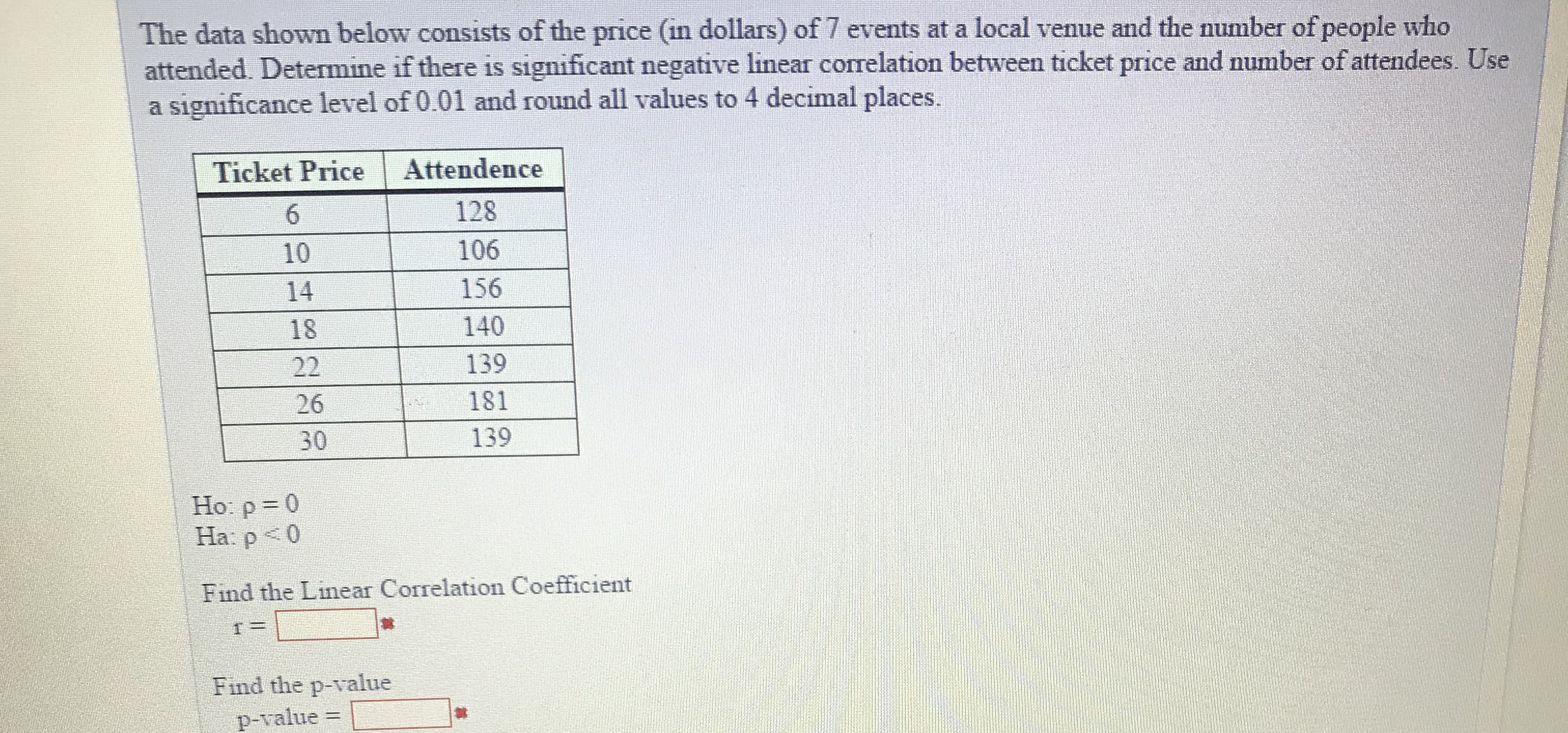# The data shown below consists of the price (in dollars) of 7 events at a local venue and the number of people whoattended. Determine if there is significant negative linear correlation between ticket price and number of attendees. Usea significance level of 0.01 and round all values to 4 decimal places.Ticket Price Attendence10182612810615614013918113930Ho: p=0Ha: poFind the Linear Correlation CoefficientFind the p-value韎p-value

Question
25 viewshelp_outlineImage TranscriptioncloseThe data shown below consists of the price (in dollars) of 7 events at a local venue and the number of people who attended. Determine if there is significant negative linear correlation between ticket price and number of attendees. Use a significance level of 0.01 and round all values to 4 decimal places. Ticket Price Attendence 10 18 26 128 106 156 140 139 181 139 30 Ho: p=0 Ha: po Find the Linear Correlation Coefficient Find the p-value 韎 p-value fullscreen
check_circle

Step 1

In this question, we have the data about Ticket Price and Attendance, we have to find the correlation coefficient between these two variables and then we have to conduct the hypothesis testing.

Step 2

Here I have calculated the correlation coefficient and for the calculation of correlation coefficient we had calculated sum value that has shown in table mentioned on whiteboard and the calculation for correlation coefficient has also been mentioned on whiteboard.

Step 3

Now here we have conducted the hypothesis testing and calc...

### Want to see the full answer?

See Solution

#### Want to see this answer and more?

Solutions are written by subject experts who are available 24/7. Questions are typically answered within 1 hour.*

See Solution
*Response times may vary by subject and question.
Tagged in

### Hypothesis Testing